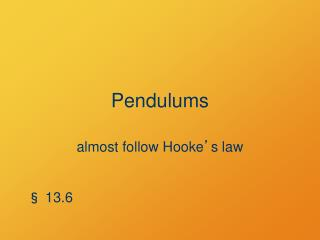DownloadDownload PresentationPendulums

# Pendulums

Download Presentation## Pendulums

- - - - - - - - - - - - - - - - - - - - - - - - - - - E N D - - - - - - - - - - - - - - - - - - - - - - - - - - -
##### Presentation Transcript

1. Pendulums almost follow Hooke’s law § 13.6

2. Angular Oscillators • Angular Hooke’s law: t = –kq • Angular Newton’s second law: t = Ia • So –kq =Ia • General Solution: q = Q cos(wt + f) • where w2 = k/I; Q and f are constants

3. Simple Pendulum L q m • Massless, inextensible string/rod • Point-mass bob

4. Poll Question The period of a simple pendulum depends on: (Add together the numbers for all correct choices and enter the sum.) 1. The length L. 2. The mass m. 4. The maximum amplitude Q. 8. The gravitational field g.

5. L T = wR + mv2/L q wR = mg cosq q w = mg m wT = mg sinq Simple Pendulum Force SFT = –wT = –mg sinq

6. Simple Pendulum Torque SFT= –wT = –mg sinq = LFT = –L mg sinq Restoring torque L q m

7. Small-Angle Approximation For small q (in radians) q sin q  tan q

8. Simple Pendulum t = –L mg sinq t  –L mg q = –kq k = Lmg I = mL2 L q m Lmg mL2 w2 = k/I = = g/L w is independent of mass m (w is not the angular speed of the pendulum)

9. Board Work Find the length of a simple pendulum whose period is 2 s. About how long is the pendulum of a grandfather clock?

10. Think Question An extended object with its center of mass a distance L from the pivot, has a moment of inertia • greater than • the same as • less than a point mass a distance L from the pivot.

11. Poll Question If a pendulum is an extended object with its center of mass a distance L from the pivot, its period is • longer than • the same as • shorter than The period of a simple pendulum of length L.

12. Physical Pendulum Source: Young and Freedman, Figure 13.23.

13. w = k mgd = I I Physical Pendulum Fnet = –mg sinq tnet = –mgd sinq Approximately Hooke’s law t –mgdq I = Icm + md2

14. Example: Suspended Rod Mass M, center of mass at L/2 Physical pendulum Simple pendulum L L L 2 2 1 1 3 4 I =    ML2 I =    ML2 harder to turn easier to turn

15. Damped and Forced Oscillations Introducing non-conservative forces § 13.7–13.8

16. Damping Force Such as viscous drag v Drag opposes motion: F = –bv

17. Poll Question How does damping affect the oscillation frequency? Damping increases the frequency. Damping does not affect the frequency. Damping decreases the frequency.

18. Light Damping –bt 2m x(t) = Ae cos(w't + f) k – b2 w' = m 4m2 • If w' > 0: • Oscillates • Frequency slower than undamped case • Amplitude decreases over time

19. k – b2 w' = m 4m2 Critical Damping If w' = 0: x(t) = (C1 + C2t) e–at • No oscillation • If displaced, returns directly to equilibrium

20. k – b2 w' = If w' is imaginary: x(t) =C1 e–at + C2 e–a t m 4m2 1 2 Overdamping • No oscillation • If displaced, returns slowly to equilibrium

21. = F·v = –bv·v Energy in Damping • Damping force –bv is not conservative • Total mechanical energy decreases over time = –bv2 • Power

22. Forced Oscillation Periodic driving force F(t) = Fmax cos(wdt)

23. Forced Oscillation If no damping If wd = w', amplitude increases without bound

24. Critical or over-damping (b ≥ 2 km): no resonance Resonance If lightly damped: greatest amplitude when wd = w' Source: Young and Freedman, Fig. 13.28